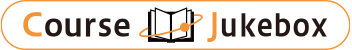### CourseDetailDegree
Master
1・2
Course delivery methods
Online (Asynchronous)
Subject
Physical sciences, Mathematical sciences, Computer Science, Engineering & technology
Program
School
Degree Programs in Systems and Information Engineering
Department
Master's Program in Risk and Resilience Engineering
Campus
Tsukuba Campus
Classroom
Course Offering Year
2022-2023
Course Offering Month
April - June
Weekday and Period
Tue5,6
Capacity
Credits
2.0
Language
English
Course Number
0AL0304

### Advanced Course on Mathematical Model Analysis University of Tsukuba

#### Course Overview

This lecture introduces a risk verification method by numerical computations of nonlinear mathematical models and a rigorous numerical method to control the risk in numerical computations. Representing
phenomena by mathematical models and predicting them by computer simulations are widely used methods for analyzing phenomena, but if the reproducibility of phenomena by mathematical models cannot be verified, unexpected risks are inherent in the analysis of phenomena. In this lecture, as a familiar method of verifying the reliability of mathematical models, each method of simulation using numerical computation is introduced. In particular, we focus on the errors in numerical computation, and discuss "rigorous numerics", which is a numerical computation method that gives correct results by taking account of all errors that occur in numerical computation.

#### Learning Achievement

The following topics will be outlined in each lecture. In order to deepen the understanding of the contents of the lecture, exercises with report assignments and group work by students will be conducted as needed.

#### Competence

The general competence of the degree program is related to "1. ability to utilize knowledge," the specialized competence of the degree program is related to "1. basic engineering skills," "2. knowledge of basic theories and related technologies," "3. knowledge of actual problems," and "4. broad perspective and bird's eye view," and the research group competence is related to "1. The research group competence is related to "1. research ability" and "2. expertise.

#### Course prerequisites

Students will be judged on a 1:2 ratio of performance on the exercises and the final exam. Students who pass the exam with an overall score of 60% or higher are considered to be successful.

Lectures

#### Instructor

Takayasu Akitoshi

#### Other information

Identical to 01CF407.
Online(Asynchronous) In this lecture, we try to bridge the gap from the basic knowledge to the state-of-the-art research results, so that the students can learn the analysis methods of mathematical models using numerical computation with their hands. We will start from the basic knowledge and sublimate the topics to the most advanced research subjects.